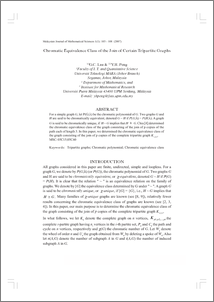# Chromatic equivalence class of the join of certain tripartite graphs

## Citation

Lau, Gee Choon and Peng, Yee Hock (2007) Chromatic equivalence class of the join of certain tripartite graphs. Malaysian Journal of Mathematical Sciences, 1 (1). pp. 103-108. ISSN 1823-8343

## Abstract

For a simple graph G, let P(G;λ) be the chromatic polynomial of G. Two graphs G and H are said to be chromatically equivalent, denoted G ~ H if P(G;λ) = P(H;λ). A graph G is said to be chromatically unique, if H ~ G implies that H ≅ G. Chia  determined the chromatic equivalence class of the graph consisting of the join of p copies of the path each of length 3. In this paper, we determined the chromatic equivalence class of the graph consisting of the join of p copies of the complete tripartite graph K1,2,3. MSC: 05C15;05C60Preview
PDF
page_103-108.pdfView Item